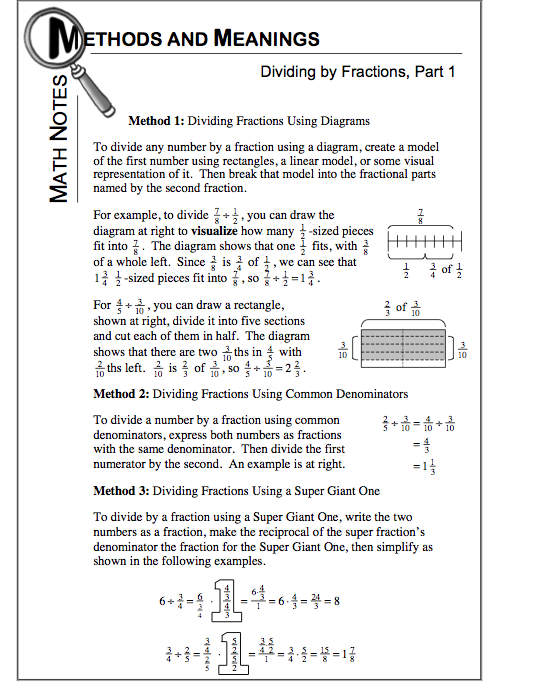### Home > MC1 > Chapter 9 > Lesson 9.2.5 > Problem9-112

9-112.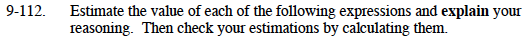For parts (a) through (d), try rounding the fractions mentally to the nearest quarter, half, or whole number.
Then, simplify the expression mentally as well.
Don't forget to explain your reasoning!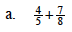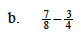To add and subtract fractions for parts (a) and (b), refer to the Math Notes box below.

$\text{The actual difference is }\frac{1}{8}. \text{ Did you find the answer to part (a)?}$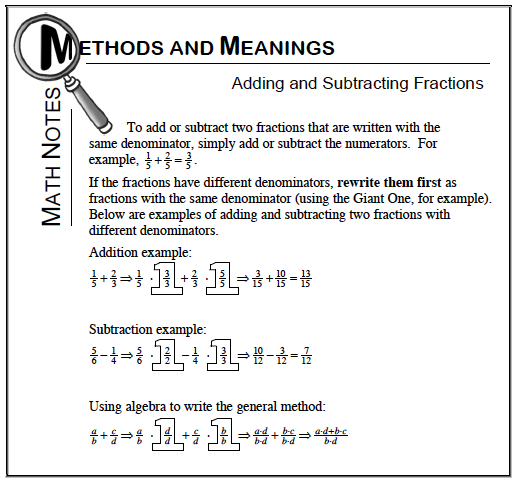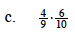Remember, to multiply fractions, you can multiply the top numbers (numerators) together and the bottom numbers (denominators) together.
Don't forget to make an estimate first!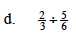$\text{How many }\frac{5}{6} \text{ fit into }\frac{2}{3}?$

More than one? Less than one? Why?
$\text{The actual quotient is }\frac{4}{5}.$